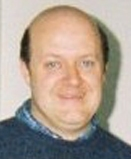Enter the content which will be displayed in sticky bar

# MemberMichael Harney
local time: 2023-01-29 05:32 (-06:00 DST)
Michael Harney (Abstracts)
Titles
• [Updated 6 years ago]
• [Updated 6 years ago]
• [Updated 6 years ago]
• [Updated 6 years ago]
Abstracts Details
• [Updated 6 years ago]

In the study of an Earth orbiting satellite, the terms of the series expansion of the Earth's gravitational potential can be expressed as functions of the eccentricity of the satellite. These functions are also known as eccentricity functions. The series expansion of these functions given by Kaula  appears to result in instabilities at high eccentricities. When calculating the eccentricity functions, researchers resort to numerical integration techniques instead. The approach followed in this contribution bypasses the problem of instability at high eccentricities by using a Hansen coefficient definition. As a test, we first calculate analytical expressions for various known eccentricity functions and then we proceed with the calculation of the eccentricity functions associated with degree and order 20, 30, 40, 50 sectorial harmonic coefficient expansion of the gravitational potential. Our calculation demonstrates the efficiency of Hansen coefficient approach that differs from that given by Kaula. It is efficient, fast, and can easily be performed with the help of a personal computer, with no instabilities at higher eccentricities.

Recent observations confirm that galactic red-shifts might be quantized and hint a possible
new form of quantum mechanics, which could probably explain these observed
properties of the galaxies. This brief note investigates some expressions for the mass of
the universe M(u), which were obtained with the help of the definition of the new cosmic
Planck constant h bar subscript g.

The SRT frequency shift, time dilation and energy equivalence formulas are derived from wave structure of matter concepts. The derivation shown removes Einstein?s requirement for the constancy of the speed of light between reference frames, which is not necessary in producing the results of the Lorentz transforms. Also, the photon is revealed to be the interaction between two electron wave centers, not a separate particle, showing a preference for Tetrode's concept of a photon.

• [Updated 6 years ago]

Schwarzschild's g44 solution for time dilation and frequency shift of light are derived from wave structure of matter concepts as opposed to General Relativity. This corroborates the origin of time and frequency shifts predicted by the wave structure of matter and the nature of a photon. This derivation relates known values with the properties of the Universe, and shows the origin of the Newtonian gravitation constant.

• [Updated 6 years ago]

It is shown that if space is modeled as an elastic medium that propagates spherical, scalar quantum-waves, then the ratio of the square of the wave velocity to c2 reveals the same results as the familiar time dilation formula that is produced from the Schwarzchild G44 component. The Schwarzchild radius derived from the scalar-wave model is shown to be equal to the radius of the universe, implying that there are no gravitational singularities present within the radius of the Universe.

Using a non-singular gravitational potential which appears in the literature we analytically
derived and investigated the equations describing the precession of a body?s spin
orbiting around a main spherical body of mass M. The calculation has been performed
using a non-exact Schwarzschild solution, and further assuming that the gravitational
field of the Earth is more than that of a rotating mass. General theory of relativity predicts
that the direction of the gyroscope will change at a rate of 6.6 arcsec/year for a
gyroscope in a 650 km high polar orbit. In our case a precession rate of the spin of a
very similar magnitude to that predicted by general relativity was calculated resulting
to a (Delta)Sgeo / Sgeo = -5.570x10-2.

• [Updated 6 years ago]

General Relativity predicts the existence of relativistic corrections to the static Newtonian potential which can be calculated and verified experimentally. The idea leading to quantum corrections at large distances is that of the interactions of massless particles which only involve their coupling energies at low energies. In this short paper we attempt to propose the Sagnac intrerferometric technique as a way of detecting the relativistic correction suggested for the Newtonian potential, and thus obtaining an estimate for phase di
erence using a satellite orbiting at an altitude of 250 km above the surface of the Earth.